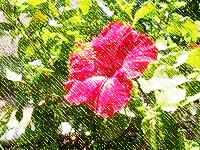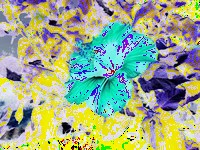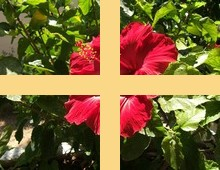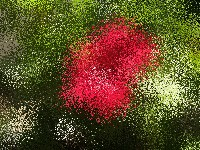# Rubblewebs

THESE CODES ARE PROVIDED FOR AN EXAMPLE OF HOW TO USE IMAGEMAGICK WITH PHP. CARE SHOULD BE TAKEN WHEN ACCEPTING USER INPUT.

I TAKE NO RESPONSABILTY FOR ANY PROBLEMS THAT MAY OCCURE WHEN USING ANY OF THIS CODE.

IT IS UP TO YOU TO SECURE THE CODE AND VALIDATE USER INPUT.

# Imagick Functions page: 22

## Set type

( Sets the image type attribute. )

Shines a distant light on an image to create a three-dimensional effect.

( You control the positioning of the light with azimuth and elevation; azimuth is measured in degrees off the x axis and elevation is measured in pixels above the Z axis. )```\$im = new Imagick(\$input);
\$shadow->setImageBackgroundColor( new ImagickPixel( 'black' ) );
\$shadow->compositeImage( \$im, Imagick::COMPOSITE_OVER, 0, 0 );
```

## Sharpen image

Sharpens an image.```\$im = new Imagick(\$input);
\$im->sharpenImage( 2, 0);
\$im->writeImage('sharpenImage.jpg');
\$im->destroy();
```

( We convolve the image with a Gaussian operator of the given radius and standard deviation (sigma). For reasonable results, the radius should be larger than sigma. Use a radius of 0 and selects a suitable radius for you. )

## Shave image

Shaves pixels from the image edges.```\$im = new Imagick(\$input);
\$im->shaveImage( 5, 50 );
\$im->writeImage('shaveImage.jpg');
\$im->destroy();
```

( It allocates the memory necessary for the new Image structure and returns a pointer to the new image. )

## Shear image

Slides one edge of an image along the X or Y axis, creating a parallelogram.```\$im = new Imagick(\$input);
\$im->shearImage( new ImagickPixel( 'none' ), 10, 0 );
\$im->writeImage('shearImage.png');
\$im->destroy();```

( An X direction shear slides an edge along the X axis, while a Y direction shear slides an edge along the Y axis. The amount of the shear is controlled by a shear angle. For X direction shears, x_shear is measured relative to the Y axis, and similarly, for Y direction shears y_shear is measured relative to the X axis. Empty triangles left over from shearing the image are filled with the background color. )

## sigmoidalContrastImage

Adjusts the contrast of an image with a non-linear sigmoidal contrast algorithm.```\$im = new Imagick(\$input);
\$im->sigmoidalContrastImage( 0.8, 1, 1 );
\$im->writeImage('sigmoidalContrastImage.jpg');
\$im->destroy();
```

( Increase the contrast of the image using a sigmoidal transfer function without saturating highlights or shadows. Contrast indicates how much to increase the contrast (0 is none; 3 is typical; 20 is pushing it); mid-point indicates where midtones fall in the resultant image (0 is white; 50 is middle-gray; 100 is black). Set sharpen to TRUE to increase the image contrast otherwise the contrast is reduced. )

## Sketch image

Simulates a pencil sketch.```\$im = new Imagick(\$input);
\$im->sketchImage( 10, 0, 45 );
\$im->writeImage('sketchImage.jpg');
\$im->destroy();
```

( We convolve the image with a Gaussian operator of the given radius and standard deviation (sigma). For reasonable results, radius should be larger than sigma. Use a radius of 0 and Imagick::sketchImage() selects a suitable radius for you. Angle gives the angle of the blurring motion. )

## solarizeImage

Applies a special effect to the image, similar to the effect achieved in a photo darkroom by selectively exposing areas of photo sensitive paper to light.```\$im = new Imagick(\$input);
\$im->solarizeImage( 2000 );
\$im->writeImage('solarizeImage.jpg');
\$im->destroy();
```

( Threshold ranges from 0 to QuantumRange and is a measure of the extent of the solarization. )

## Sparse color image

Interpolates colors

Untested - documentation seems wrong for the array.

```/*
\$new->sparseColorImage( Imagick::SPARSECOLORMETHOD_BARYCENTRIC, array( 0, 0, black, 0, 20, white ) );
*/```

( Given the arguments array containing numeric values this method interpolates the colors found at those coordinates across the whole image using sparse_method. )

## Splice image

Splices a solid color into the image```\$im = new Imagick(\$input);
\$im->spliceImage( 20, 20, 100, 75 );
\$im->writeImage('spliceImage.jpg');
\$im->destroy();
```

Special effects method that randomly displaces each pixel in a block defined by the radius parameter.```\$im = new Imagick(\$input);